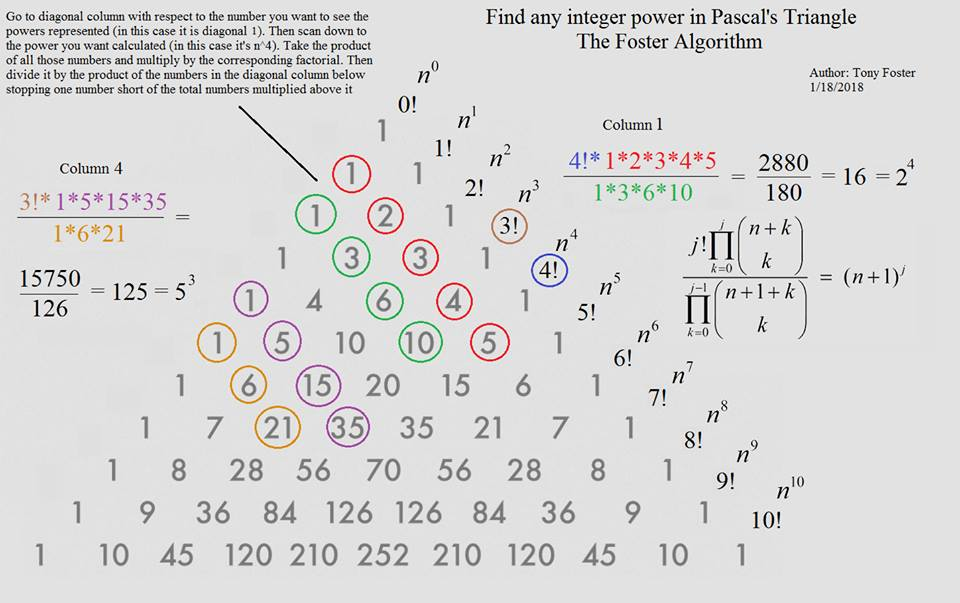# Tony Foster's Integer Powers in Pascal's Triangle

### Source### Problem

For integer $n,j\gt 1,$ we have

$\displaystyle \fracj!\prod_{k=0}^{j}{n+k\choose k}}\prod_{k=0}^{j-1}{n+1+k\choose k}}=(n+1)^j$

Note that the multiplicands in the two products come from successive rows (or columns) of Pascals's triangle.

### Solution 1

We start with an obvious $\displaystyle {n+1+k\choose k}=\frac{n+1+k}{n+1}{n+k\choose k}.$ Using that,

\displaystyle \begin{align} \fracj!\prod_{k=0}^{j}{n+k\choose k}}\prod_{k=0}^{j-1}{n+1+k\choose k}}&=\fracj!\prod_{k=0}^{j-1}{n+k\choose k}\cdot {n+j\choose j}}\prod_{k=0}^{j-1}\frac{n+1+k}{n+1}\cdot\prod_{k=0}^{j-1}{n+k\choose k}}\\ &=\fracj!{n+j\choose j}}\prod_{k=0}^{j-1}\frac{n+1+k}{n+1}}=\frac{(n+j)!}{n!}\cdot\frac(n+1)^j}{\displaystyle\prod_{k=0}^{j-1}(n+1+k)}\\ &=\frac{(n+1)^j\cdot (n+j)!}{(n+j)!}=(n+1)^j. \end{align

### Solution 2

We'll use induction on $j.$ Let $\displaystyle F(j)=\fracj!\prod_{k=0}^{j}{n+k\choose k}}\prod_{k=0}^{j-1}{n+1+k\choose k}$ and assume $F(m)=(n+1)^m,$ for some $m\gt 0.$ Then

\displaystyle \begin{align} \frac{F(m+1)}{F(m)}&=\frac(m+1){n+m+1\choose m+1}}{n+1+m\choose m}}\\ &= \frac{(m+1)(n+m+1)!}{n!(m+1)!}\cdot\frac{(n+1)!m!}{(n+1+m)!}=n+1, \end{align

implying $F(m+1)=F(m)(n+1)=(n+1)^m(n+1)=(n+1)^{m+1}.$

### Acknowledgment

This is Tony Foster's invention that he kindly shared on the CutTheKnotMath facebook page. Solution 1 is a slight modification of his original proof.

The above is a generalization of Tony's previous discovery.

### Pascal's Triangle and the Binomial Coefficients